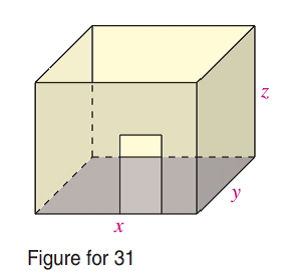Chapter 7.6, Problem 31E### Calculus: An Applied Approach (Min...

10th Edition
Ron Larson
ISBN: 9781305860919

#### Solutions

Chapter
Section### Calculus: An Applied Approach (Min...

10th Edition
Ron Larson
ISBN: 9781305860919
Textbook Problem
1 views

# Cost In redecorating an office, the cost for new carpeting is $4 per square foot and the cost of wallpapering a wall is$3 per square foot. Find the dimensions of the largest office that can be redecorated for $1728 (see figure). (Hint: Maximize V = x y z subject to 4 x y + 6 x z + 6 y z = 1728. )To determine To calculate: The dimensions of the largest office than can be redecorated for$1728 when in redecorating an office, the cost for new carpeting is $4 per square foot and the cost for wallpapering a wall is$3 per square foot.

Explanation

Given Information:

The structure of office is provided in the image below as,

The cost for new carpeting is $4 per square foot and the cost for wallpapering a wall is$3 per square foot.

Formula used:

The volume of the cuboid V is given as,

V=xyz

Where x, y and z is the length, breadth and height of the cuboid.

Method of Lagrange multipliers,

If the function f(x,y) contains a maximum or minimum subject to the constraint g(x,y)=0 then the maximum or minimum can occur at one of the critical numbers of the function F is,

F(x,y,λ)=f(x,y)λg(x,y) where, λ is a Lagrange multiplier.

Steps to determine the minimum or maximum of the function f.

1. Solve the system of equations,

Fx(x,y,λ)=0Fy(x,y,λ)=0Fλ(x,y,λ)=0

2. Determine the value of the function f at each solution obtained from the step 1.

The largest value gives the maximum value of function f subject to the constraint g(x,y)=0 and the lowest value gives the minimum value of function f subject to the constraint g(x,y)=0.

Calculation:

Let x, y and z be the length, breadth and height of the cuboid shaped office, then volume would be,

V=xyz

So, the function would be considered as, f(x,y,z)=xyz.

Now find the subject to constraint equation.

The floor area would be, xy and the wall area would be 2(xz+yz).

Since, the cost for new carpeting is $4 per square foot and the cost for wallpapering a wall is$3 per square foot and the total cost is \$1728.

So, the equation would be formed as,

4(floor area)+3(wall area)=Total cost4xy+6xz+6yz=1728

Thus, the subject to constraint equation would be 4xy+6xz+6yz=1728.

So, g(x,y)=4xy+6xz+6yz1728

Now, the new function F is,

F(x,y,z,λ)=xyzλ(4xy+6xz+6yz1728)

Now, differentiate with respect to x, y, z and λ, and then set each partial derivative equal to zero and solve as,

Fx(x,y,z,λ)=0yzλ(4y+6z)=0

And,

Fy(x,y,z,λ)=0xzλ(4x+6z)=0

And,

Fz(x,y,z,λ)</

### Still sussing out bartleby?

Check out a sample textbook solution.

See a sample solution

#### The Solution to Your Study Problems

Bartleby provides explanations to thousands of textbook problems written by our experts, many with advanced degrees!

Get Started

#### What does the Squeeze Theorem say?

Single Variable Calculus: Early Transcendentals, Volume I

#### In Exercises 49-62, find the indicated limit, if it exists. 54. limb3b+1b+3

Applied Calculus for the Managerial, Life, and Social Sciences: A Brief Approach

#### 31. Solve for t.

Mathematical Applications for the Management, Life, and Social Sciences

#### Multiply the following numbers. 508.02.61_

Contemporary Mathematics for Business & Consumers

#### The curvature of at t = 0 is:

Study Guide for Stewart's Multivariable Calculus, 8th